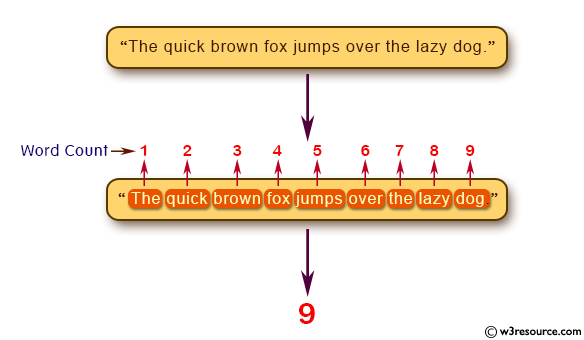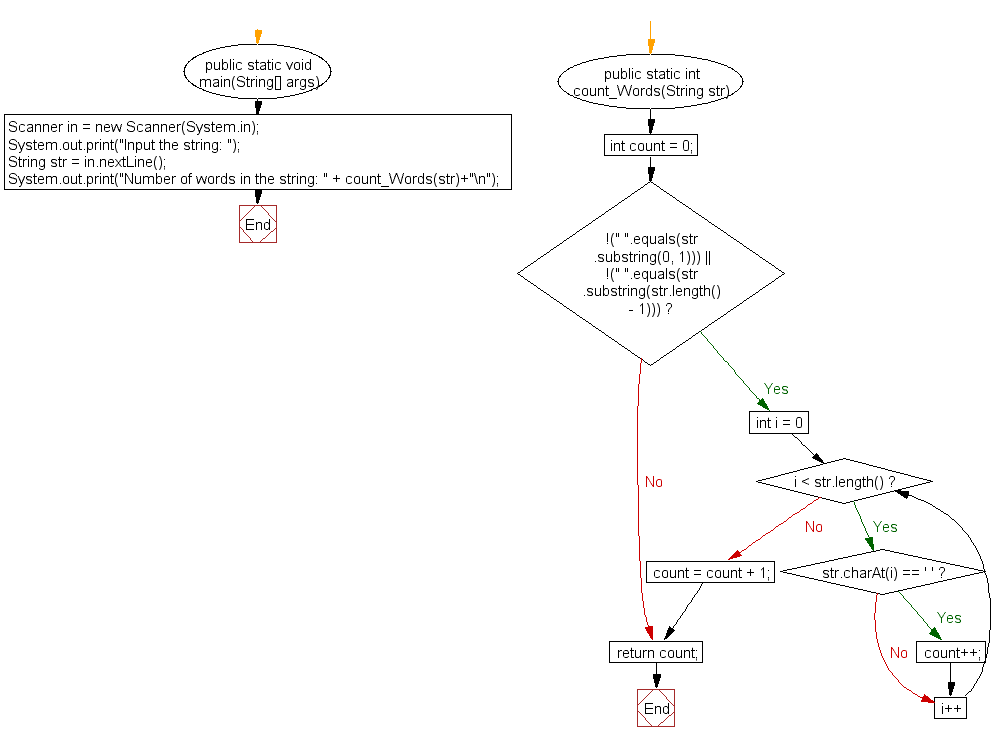﻿ Java exercises: Count all words in a string - w3resource# Java Method Exercises: Count all words in a string

## Java Method: Exercise-5 with Solution

Write a Java method to count all words in a string.

Test Data:
Input the string: The quick brown fox jumps over the lazy dog.

Pictorial Presentation:Sample Solution:

Java Code:

``````import java.util.Scanner;
public class Exercise5 {

public static void main(String[] args)
{
Scanner in = new Scanner(System.in);
System.out.print("Input the string: ");
String str = in.nextLine();

System.out.print("Number of words in the string: " + count_Words(str)+"\n");
}

public static int count_Words(String str)
{
int count = 0;
if (!(" ".equals(str.substring(0, 1))) || !(" ".equals(str.substring(str.length() - 1))))
{
for (int i = 0; i < str.length(); i++)
{
if (str.charAt(i) == ' ')
{
count++;
}
}
count = count + 1;
}
return count; // returns 0 if string starts or ends with space " ".
}
}
```
```

Sample Output:

```Input the string: The quick brown fox jumps over the lazy dog
Number of words in the string: 9
```

Flowchart:Java Code Editor: